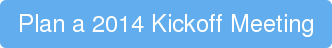Let's pretend that you need to throw a once in a lifetime culinary spectacle in Panama. If you're @ashinyknife, this will be no problem. Let's pretend you decide upon a monopoly theme. Generally, N guests start out on go, roll dice and end up on a monopoly square. Let's pretend that each square has a wholy different gastronomic creation on it. Given the above, how many cornish game hens should we expect to buy for St Charles place? How much caviar will we need to supply the B&O railroad? These are the important questions that we will set out to answer today.

## Probability

Our first approach might look something like this: http://statistics.about.com/od/ProbHelpandTutorials/a/Probability-And-Monopoly.htm Basic probability, round 1 is reasonable. Round 2 makes sense.. oh gawd round 3 starts to get hard to keep track of.

## Monte Carlo

So what should we do? It seems to me that the appropriate technique to use here is Monte Carlo simulation. What is Monte Carlo? Honestly Monte Carlo should be pretty attractive to those of us for whom probability 101 was a long time ago. Basically "Monte Carlo simulation" means "let's just see what really happens". Say I ask you to figure out the probability that when flipping a coin 100 times I get at least one run of 10 heads. You've got two choices: 1) Figure out the appropriate math. 2) Flip a coin 100 times. Figure out if you get 10 heads in a row. Do this 1 million times and calculate the percentage of times when it was true. Option 2 is monte carlo.

## Time for computers

This is really pretty easy to code up. Create a two-dimension array. Dimension one will keep track of each simulation. Dimension two will track each of the 40 Monopoly squares.
For each simulation, for each user in the simulation, for each of the moves, move them around the board.
To move them around the board we just roll two dice, and move us along.
Finally it's just a matter of averaging up the values for each square in our simulation and voila

## Summary

So now the big question: Did we answer our original question? Do we know how much food to buy? Say we're planning on serving 4 courses. Do we feel figuring out how many hens we would need for an even distribution, then buying 5.44x the cornish game hens for 'Chance' and 3.8x the caviar for the B&O railroad?

## What do you think?

See my answer in The Statistics of Monopoly with Respect to Cornish Game Hen Provisioning: Part 2 "Probability is a bitch"